# Samacheer Kalvi 8th Maths Solutions Term 2 Chapter 2 Algebra Ex 2.4

Students can Download Maths Chapter 2 Algebra Ex 2.4 Questions and Answers, Notes Pdf, Samacheer Kalvi 8th Maths Book Solutions Guide Pdf helps you to revise the complete Tamilnadu State Board New Syllabus and score more marks in your examinations.

## Samacheer Kalvi 8th Maths Solutions Term 2 Chapter 2 Algebra Ex 2.4

Question 1.
Fill in the blanks:

Question (i)
y = px where p ∈ Z always passes through the ………
Origin (0, 0)
Hint:
[When we substitute x = 0 in equation, y also becomes zero. (0,0) is a solution]Question (ii)
The intersecting point of the line x = 4 and y = – 4 is ………
4, -4
Hint:
x = 4 is a line parallel to the y – axis and
y = – 4 is a line parallel to the x – axis. The point of intersection is a point that lies on both lines & which should satisfy both the equations. Therefore, that point is (4, -4)

Question (iii)
Scale for the given graph, on the x – axis 1 cm = ……… units y – axis 1 cm = ………. units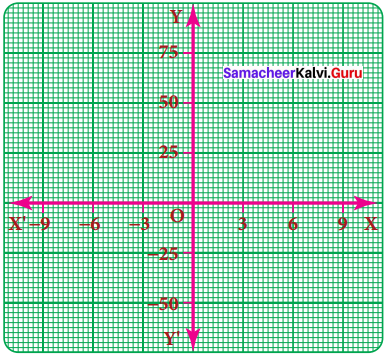3 units, 25 units
Hint:
With reference to given graph,
On the x – axis, 1cm = 3 units
y axis, 1cm = 25 units

Question 2.
Say True or False:

Question (i)
The points (1, 1) (2, 2) (3, 3) lie on a straight line.
True
Hint:
The points (1, 1), (2, 2), (3, 3) all satisfy the equation y = x
which is straight line. Hence, it is trueQuestion (ii)
y = – 9x not passes through the origin.
False
Hint:
y = – 9x substituting forx as zero, we get y = – 9 x 0 = 0
∴ for x = 0, y = 0. Which means line passes through (0, 0), hence statement is false.

Question 3.
Will a line pass through (2, 2) if it intersects the axes at (2, 0) and (0, 2).
Solution:
Given a line intersects the axis at (2, 0) & (0, 2)
Let line intercept form be expressed as
ax + by = 1 Where a & b are the x & y intercept respectively.
Since the intercept points are (2, 0) & (0, 2)
a = 2, b = 2
∴ 2x + 2y = 1
When the point (2, 2) is considered & substituted in the equation
2x + 2y = 1 we get
2 x 2 + 2 x 2 = 4 ≠ 1
∴ The point (2, 2) does not satisfy the equation. Therefore the line does not pass through (2, 2)
Alternatively graphical method:
as we can see the line doesn’t pass through (2, 2)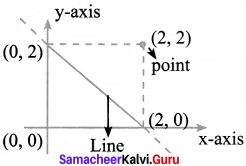Question 4.
A line passing through (4, – 2) and intersects the Y – axis at (0, 2). Find a point on the line in the second quadrant.
Solution: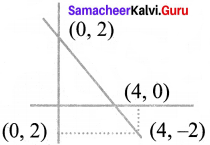Line passes through (4, – 2)
y – axis intercept point – (0, 2)
using 2 point formula,
$$\frac { y-y_{ 1 } }{ x-x_{ 1 } }$$ = $$\frac { { y }_{ 2 }-{ y }_{ 1 } }{ { x }_{ 2 }-{ x }_{ 1 } }$$
$$\frac{y-2}{x-0}$$ = $$\frac{1-2-2}{4-0}$$
∴ $$\frac{y-2}{x}$$ = $$\frac{-4}{4}$$ = – 1
y – 2 = – 1 × x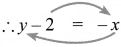∴ x + y = 2 is the equation of the line.
Any point in II quadrant will have x as negative & y as positive.
So let us take x value as – 2
∴ – 2 + y = 2
y = 2 + 2 = 4
∴ Point in II Quadrant is (-2, 4)Question 5.
If the points P (5, 3) Q(- 3, 3) R (- 3, – 4) and S form a rectangle then find the coordinate of S.
Solution:
Plotting the points on a graph (approximately)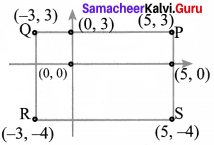Steps:

1. Plot P, Q, R approximately on a graph.
2. As it is a rectangle, RS should be parallel to PQ & QR should be parallel to PS
3. S should lie on the straight line from R parallel to x – axis & straight line from P parallel to y – axis
4. Therefore, we get S to be (5, -4)

[Note: We don’t need graph sheet for approximate plotting. This is just for graphical understanding]

Question 6.
A line passes through (6, 0) and (0, 6) and an another line passes through (- 3, 0) and (0, – 3). What are the points to be joined to get a trapezium?
Solution:
In a trapezium, there are 2 opposite sides that are parallel. The other opposite sides are non-parallel.
Now, let us approximately plot the points for our understanding [no need of graph sheet]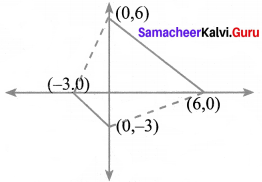1. Plot the points (0, 6), (6, 0), (-3, 0) & (0, – 3)
2. Join (0, 6) & (6, 0)
3. Join (-3, 0) & (0, -3)
4. We find that the lines formed by joining the points are parallel lines.
5. So, for forming a trapezium, we should join (0, 6), (-3, 0) & (0, -3), (6, 0)

Question 7.
Find the point of intersection of the line joining points (- 3, 7) (2, – 4) and (4, 6) (- 5, 7). Also find the point of intersection of these lines and also their intersection with the axis.
Solution: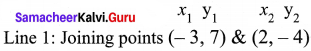Equation of line joining 2 points by 2 point formula is given by
$$\frac { y-y_{ 1 } }{ x-x_{ 1 } }$$ = $$\frac { { y }_{ 2 }-{ y }_{ 1 } }{ { x }_{ 2 }-{ x }_{ 1 } }$$
∴ $$\frac{y-7}{x-(-3)}$$ = $$\frac{-4-7}{2-(-3)}$$
$$\frac{y-7}{x+3}$$ = $$\frac{-11}{2+3}$$
∴ $$\frac{y-7}{x+3}$$ = $$\frac{-11}{5}$$
Cross multiplying, we get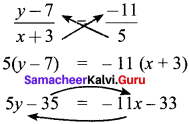Transposing the variables, we get
11x + 5y = 35 – 33 = 2
11x + 5y = 2 – Line 1
Similarly, we should find out equation of second line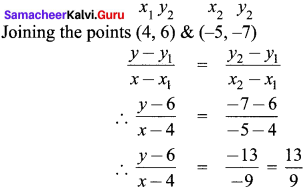∴ 9y – 54 = 13x – 52
9y – 13x = 2 – Line 2
For finding point of intersection, we need to solve the 2 line equation to find a point that will satisfy both the line equations.
∴ Solving for x & y from line 1 & line 2 as below
11x + 5y = 2 ⇒ multiply both sides by 13,
11 x 13x + 5 x 13y = 26
Line 2:
9y – 13x = 2 ⇒ multiply both sides by 11
9 x 11y – 13 x 11x = 22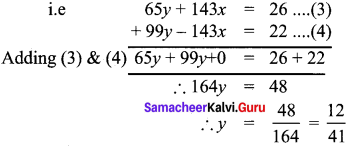Substituting this value of y in line 1 we get
11x + 5y = 2
11x + 5 x $$\frac{12}{41}$$ = 2
11x = 2 – $$\frac{60}{41}$$ = $$\frac{82-60}{41}$$ = $$\frac{22}{41}$$
x = $$\frac{2}{41}$$
∴ Point of intersection is ($$\frac{2}{41}$$ $$\frac{12}{41}$$)
To find point of intersection of the lines with the axis, we should substitute values & check
Line 1:
11x + 5y = 2
Point of intersection of line with x – axis, i.e y coordinate is ‘0’
∴ put y = 0 in above equation
∴ 11x – 5 x 0 = 2
11x + 0 = 2
x = $$\frac{2}{11}$$
∴ Point is ($$\frac{2}{11}$$, 0)
Similarly, point of intersection of line with y – axis is when x – coordinate becomes ‘0’
∴ put x = 0 in above equation
∴ 11 x 0 + 5y = 2
∴ 0 + 5y = 2
y = $$\frac{2}{5}$$
∴ Point is (0, $$\frac{2}{5}$$)
Similarly for line 2,
9y – 13x = 2
For finding x intercept, i.e point where line meets x axis, we know thaty coordinate becomes ‘0’
∴ Substituting y – 0 in above eqn. we get
9 x 0 – 13x = 2
∴ 0 – 13x = 2
x = $$\frac{-2}{13}$$
∴ Point is ($$\frac{-2}{13}$$, 0)
Similarly for y – intercept, x – coordinate becomes ‘0’,
∴ Substituting for x = 0 in above equation, we get
9y – 13 x 0 = 2
9y – 0 = 2
9y = 2
y = $$\frac{2}{9}$$
Point is (0, $$\frac{2}{9}$$)Question 8.
Draw the graph of the following equations:

1. x = – 7
2. y = 6

Solution: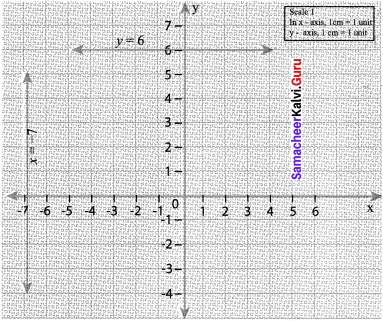Question 9.
Draw the graph of

1. y = – 3x
2. y = x – 4

Solution:
To draw graph, we need to find out some points.
1. for y = – 3x, let us first substituting values & check
put x = 0
y = – 3 x 0 = 0 ∴ (0,0) is a point
put x = 1
y = – 3 x 1 = – 3 ∴ (1, – 3) is a point
If join these 2 points, we will get the line

2. for y = x – 4
put x = 0
y = 0 – 4 = -4 ∴ (0, – 4) is a point
x = 4
y = 4 – 4 = 0 ∴ (4, 0) is a point
Now let us plot the points & join them on graph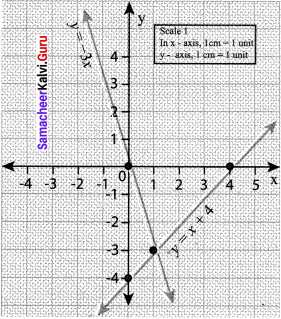Question 10.
Find the values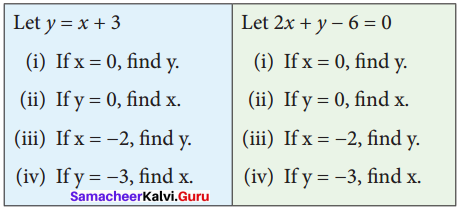Solution:
Let y = x + 3
(i) if x = 0
y = 0 + 3 = 3
∴ y = 3

(ii) y = 0
0 = x + 3
∴ x = – 3

(iii) x = – 2
y = – 2 + 3
∴ y = 1

(iv) y = -3
-3 = x + 3
∴ x = -6

Let 2x + 7 – 6 = 0
(i) x = 0
2 x 0 + y – 6 = 0
∴ 7 = 6

(ii) y = 0
2x + 0 – 6 = 0
2x = 6
x = 3

(iii) x = – 2
2 x (- 2) + y – 6 = 0
y – 10 = 0
y = 10

(iv) y = -3
2x – 3 – 6 = 0
2x = 9
x = $$\frac{9}{2}$$Question 11.
The following is a table of , values connecting the radii of a few circles and their circumferences (Taking π = $$\frac{22}{7}$$) Illustrate the relation with a graph and find

1. The radius when the circumference is 242 units.
2. The circumference when the radius is 24.5 units.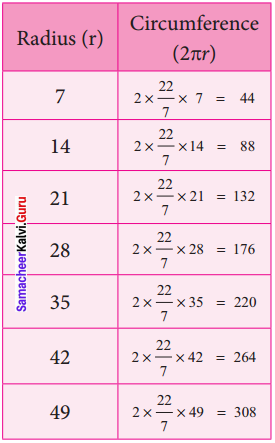Solution: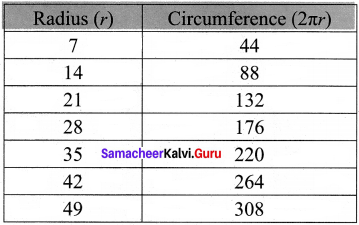r = 24.5 Circumference?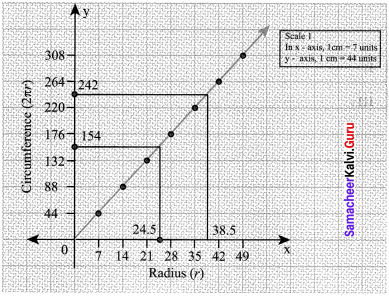Steps :

1. Draw the axis, x – axis = radius & y – axis = circumference
2. Mark the points by choosing scale of 1 cm = 7 units for x axis & 1 cm = 44 units for y – axis
3. Join the points to form a line.
4. Now for r value = 24.5 (mid value between 21 & 28), draw vertical line to touch the main line & from there drop line parallel to x axis and note where it meets y axis.

It meets between 132 & 176. Taking the mid value
i.e $$\frac{132+176}{2}$$ = 154, we get circumference of 154 when r = 24.5.
Similarly, for circumference of 242, we get r value to be 38.5

Question 12.
An over-head tank is full with water. Water leaks out from it, at a constant rate of 10 litres per hour. Draw a “time-wastage” graph for this situation and find

1. The water wasted in 150 minutes
2. The time at which 75 litres of water is wasted.

Solution:
From over head tank, water leaks out at 10 l/hr.
∴ Let us see how much leakage happens with time
Time     Leakage
1 hr        10 ltrs.
2 hrs       20 ltrs.
3 hrs       30 ltrs.
4 hrs       40 ltrs.. and so on
150 min = 120 min + 30 min
= 2 hr 30 min = 2 $$\frac{1}{2}$$ hrs
Now let us plot a graph between time & water leakage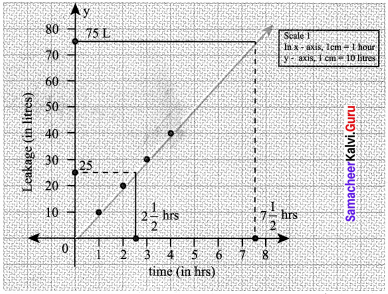Water wasted in 150 min (2$$\frac{1}{2}$$ hrs) = 25 litres
Time at which 75 litre of water is wasted = 7$$\frac{1}{2}$$ hrs = 450 minutes.